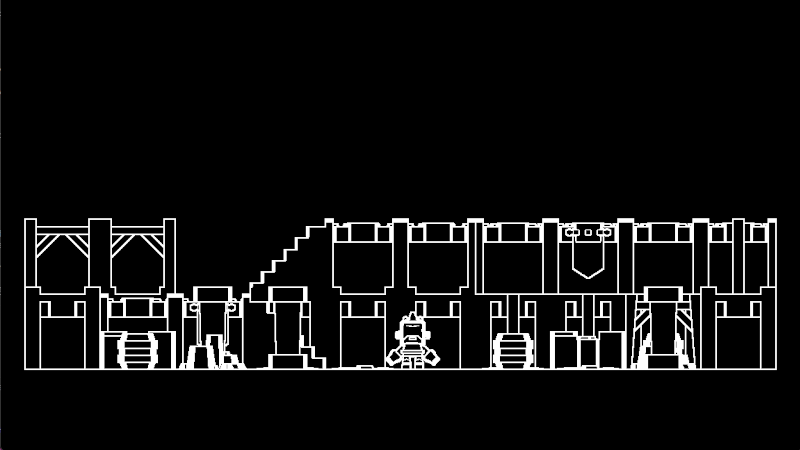# Depth-based Edge Detection with Sobel Operator – Screenspace

Spatial shader that uses the depth texture to find edges. This is a less-complex look at edge detection, perfect for learning screenspace shaders.

Instructions:

1. Create a new MeshInstance3D in your 3D scene
• Optional? Move the mesh out of the center of the screen
3. In `Geometry` for the instance, increase the `Extra Cull Margin` to the max
• As of writing, 16384 m
4. In the Quadmesh, increase the size from 1×1 m to 2×2 m
5. In the Quadmesh, check the `Flip Faces` box so that it’s `True`
8. Here you can choose a name for your shader and we can start customizing!

Notes:

This shader does an okay job at edge detection, but it has some issues.

• In a perspective camera mode, flat areas that stretch toward the horizon can get picked up as a huge edge because of how fast it falls away from the camera
• there are lots of ways to solve this issue, the one I’ve heard about most is to use the normal vectors
• basically to make sure the two pixels are on different planes
• you can get thinner lines by reducing your offset
• `vec2 offset = 0.5 / VIEWPORT_SIZE;`
• this effectively upscales your depth texture when looking at neighboring pixels
• I have been told this has an impact on performance, so be wary

Check out my full write-up here: https://github.com/nuzcraft/unga-dungeon/blob/main/shader_notes/depth_based_edge_detection_w_sobel.md

Models by Kenny – https://kenney.nl/

``````shader_type spatial;

uniform sampler2D SCREEN_TEXTURE: hint_screen_texture, filter_linear_mipmap;
uniform sampler2D DEPTH_TEXTURE: hint_depth_texture, filter_linear_mipmap;

uniform float edge_threshold = 0.1;
uniform vec3 line_color: source_color = vec3(1.0);
uniform vec3 background_color: source_color = vec3(0.0);

const mat3 sobel_y = mat3(
vec3(1.0, 0.0, -1.0),
vec3(2.0, 0.0, -2.0),
vec3(1.0, 0.0, -1.0)
);

const mat3 sobel_x = mat3(
vec3(1.0, 2.0, 1.0),
vec3(0.0, 0.0, 0.0),
vec3(-1.0, -2.0, -1.0)
);

float linearize_depth(vec2 uv_coord, mat4 proj_matrix){
float depth = texture(DEPTH_TEXTURE, uv_coord).x;
vec3 ndc = vec3(uv_coord, depth) * 2.0 - 1.0;
vec4 view = proj_matrix * vec4(ndc, 1.0);
view.xyz /= view.w;
float linear_depth = -view.z;
return linear_depth;
}

void vertex(){
POSITION = vec4(VERTEX, 1.0);
}

void fragment() {
vec2 uv = SCREEN_UV;
vec4 screen_color = texture(SCREEN_TEXTURE, uv);

float depth = linearize_depth(uv, INV_PROJECTION_MATRIX);

vec2 offset = 1.0 / VIEWPORT_SIZE;

float n = linearize_depth(uv + vec2(0.0, -offset.y), INV_PROJECTION_MATRIX);
float s = linearize_depth(uv + vec2(0.0, offset.y), INV_PROJECTION_MATRIX);
float e = linearize_depth(uv + vec2(offset.x, 0.0), INV_PROJECTION_MATRIX);
float w = linearize_depth(uv + vec2(-offset.x, 0.0), INV_PROJECTION_MATRIX);
float nw = linearize_depth(uv + vec2(-offset.x, -offset.y), INV_PROJECTION_MATRIX);
float ne = linearize_depth(uv + vec2(offset.x, -offset.y), INV_PROJECTION_MATRIX);
float sw = linearize_depth(uv + vec2(-offset.x, offset.y), INV_PROJECTION_MATRIX);
float se = linearize_depth(uv + vec2(offset.x, offset.y), INV_PROJECTION_MATRIX);

mat3 surrounding_pixels = mat3(
vec3(nw, n, ne),
vec3(w, depth, e),
vec3(sw, s, se)
);

float edge_x = dot(sobel_x, surrounding_pixels) + dot(sobel_x, surrounding_pixels) + dot(sobel_x, surrounding_pixels);
float edge_y = dot(sobel_y, surrounding_pixels) + dot(sobel_y, surrounding_pixels) + dot(sobel_y, surrounding_pixels);

float edge = sqrt(pow(edge_x, 2.0)+pow(edge_y, 2.0));

if (edge > edge_threshold) {
ALBEDO = line_color;
} else {
ALBEDO = background_color;
}
}``````
###### Tags
3d, buffer, depth, detection, edge, godot, Normal, operator, screen, ScreenSpace, Sobel, space, Spatial, textureThe shader code and all code snippets in this post are under CC0 license and can be used freely without the author's permission. Images and videos, and assets depicted in those, do not fall under this license. For more info, see our License terms.

## More from nuzcraft

### 3D Edge Detection Shader (Borderlands-Style)

Subscribe
Notify of